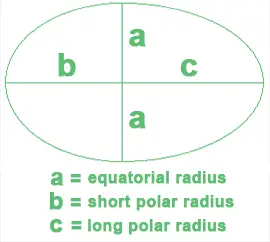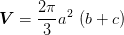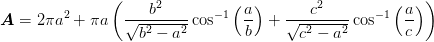# Egg Surface Area and Volume Calculator

You can use this simple egg surface area and volume calculator to determine the volume and surface area of an egg.

To compute the volume and surface area, simply input the information required in the fields below and click on the "Calculate" button to generate the results.

Egg Surface Area & Volume Calculator

units

units

units

Results
Surface Area (A) =  square units
Volume (V) =  cubic units
Surface Area to Volume Ratio (A/V) =

## The Volume of an Egg

The equatorial diameter is used to denote the width of an egg. Simply half this number to compute the egg's equatorial radius (a). The short polar radius is the distance between the equator and the nearest pole (b). The long polar radius is the distance from the egg's equator to the farthest pole (c).The shape of an egg consists of two halves of ellipsoids that have separate radius dimensions. One half has the radii of a, a, and b, while the second has the radii of a, a, and c. The volume of the egg can be calculated as follows:

V = (1/2) (4π/3) a2b + (1/2) (4π/3) a2c

= (2π/3) a2 (b + c),Where π is the constant 3.14159265.

## The Surface Area of an Egg

To calculate the surface area of an egg, you will need to use cos-1, which is the inverse trigonometric function arccos. You can calculate the surface area of an egg using the arccos button on a scientific calculator; however, make sure that the mode is on radians as opposed to degrees. The formula used to compute the surface area of an egg is as follows:## Example

Let's say we have an egg that has the following dimensions:

Equatorial radius: 2 cm (a); short polar radius: 2.5 cm (b); and long polar radius: 3.5 cm (с).

The volume of the egg is as follows:

V = (2π/3) 22 (2.5 + 3.5)

= 50.265 cm3, or 50 cm3 (rounded to 1 sig fig).

The surface area (A) is 67.773 cm2.